# Reliability Improvement in Serial Multicellular Converters Based on STATCOM Control

Reliability Improvement in Serial Multicellular Converters Based on STATCOM Control

Abdelhak DjelladSoltane Belakehal Rachid Chenni Azzeddine Dekhane

The Higher School of Industrial Technologies, P.O.BOX.218, Annaba 23000, Algeria

Department of Electrical Engineering, Brothers Mentouri University, Constantine 25000, Algeria

Corresponding Author Email:
Page:
519-528
|
DOI:
https://doi.org/10.18280/jesa.540401
23 May 2021
|
Accepted:
20 July 2021
|
Published:
31 August 2021
| Citation

OPEN ACCESS

Abstract:

FACTS devices installation is required to guarantee high energy quality and power system stability. The present paper tackles the problem of voltage control, reactive power and power factor improvement in an electric power network by one of the AC transmission devices (FACTS) of the static synchronous parallel compensator type (STATCOM), using serial multicellular converters with different levels (three to six levels). Such converters with special attention paid to the active balancing of capacitor voltages in order to generate good quality waveforms and improve the harmonic content of the output voltage. The controller's ability and functionality are evidenced through a detailed theoretical analysis. We elaborate the compensator's operating model by stating its equivalent electrical diagram representation. This is followed by a mathematical modeling and a control model based on phase shifted pulse width modulation (PS-PWM) technique to monitor reactive power flow inside the system and maintain the voltage at the common connection point (PCC) at a steady value. The proposed system simulation is carried out using Matlab Simulink environment. Finally, a presentation and a discussion of the obtained results are provided.

Keywords:

flexible AC transmission system (FACTS), STATCOM, multilevel converter, serial multicellular converters, mathematical modeling, control, PS-PWM

1. Introduction

Over the last couple of years’ electricity demand has considerably increased through industrialization and expansion. In addition to political, economic and environmental factors, new equipment such as lines, transformers, power plants... are needed. New transmission lines often fail to relieve overloaded transmission lines and provide a sufficient stability margin to diminish cascading outages and blackouts risks [1, 2]. Addressing those problems entails further enhancement of the existing electrical system control. Flexible AC transmission devices (FACTS) are suggested to allow that improved system control.

These facilities operate based upon power electronics, and they provide new solutions to address these constraints or allow for better power flow control and management. They are intended to increase the power transit, regulate the voltage, and ensure a stable dynamic power transmission network [3, 4]. Static synchronous compensators "STATCOM" are connected in parallel at critical grid points as part of FACTS compensators featuring this capability. STATCOM is the first FACTS employing voltage source converter (VSC) . This device uses high-power transistors type GTO (gate-turn-off-off) as well as IGBT (isolated-gate bipolar). This scheme has that characteristic to support voltage robustly under high disturbances, balancing asymmetric and fluctuating loads, and therefore; damping power oscillations [4, 5].

Typically, DSTATCOM deploys two-level VSCs . Nevertheless, multilevel converters are increasingly implemented across STATCOM platforms owing to their high performance when compared to two-level converters .

Their numerous advantages include improving output waveform quality, ability to run at high voltage/power conditions, and minimizing voltage stresses incurred by power switches. Additionally, they have a low dv/dt and the pulse frequency of each of the switches is lower than the apparent frequency of the voltage applied to the load [8, 9]. The multilevel converters which are frequently demonstrated in STATCOM applications are: flying capacitor multilevel converters (FCMC), diode multilevel converters (DCMC), and cascaded H-bridge multilevel converters (CHMC) .

Multi-level cascaded H-bridge converters (CHB) are the common topologies used in STATCOM implementations. CHB converters' advantages involve low switching loss, modularity, and absence of clamping diode or clamping capacitance. Alternatively, the necessity of several isolated DC sources is noted as its major drawback .

In fact, for 3-phase applications and a smaller number of levels, DCMCs are advantageous, as the capacitors are shared by the different branches . Relative to DCMC, FCMC topology has the benefit of creating multiple voltage levels with a comparatively easy work and absence of looping diodes. Compared to DCMC, FCMC further eliminates an intermediate capacitor point to each switching pair, which results in a simple converter structure .

Out of the wide range of potential PWM implementations, multilevel sine-triangle PWM is the optimized one for a flying capacitor inverter .

Nonetheless, research studies on the optimal type of PWM schemes for an MMCC using a 5L-FC as the basic sub-module have been very limited.

The key specification required for a proper PWM approach would be the ability to keep the voltages across the sub-module clamping capacitors balanced during all operating states.

A presentation of the detailed power loss assessment of submodules, built by hybrid FC and NPC converters, regardless of the modulation method, was offered by [14, 15].

The authors provided a comparative analysis of three different sine-triangle PWM schemes for an FC-based MMCC in terms of waveform performance and power losses. Based on their attained findings, the researchers  found that the PD-PWM yields worse waveform performance in terms of THD values and higher losses due to equivalent series resistance (ESR) losses of the flying capacitors resulting from high voltage fluctuation. Simultaneously, the PS-PWM yields higher switching losses owing to its high equivalent switching frequency .

Referring to the presented approach, this paper’s main aim is to implement a complex power electronics structure which consists of a multicellular converter with different levels (three, four, five and six) together with a PS-PWM control technique for managing voltage and compensating reactive energy during a grid point.

In Section II, the major component of the system will be discussed. Section III is devoted to analyzing the simulations results. The conclusion is reported in Section IV.

2. Description and Modelling

In this study, we use a conventional three-phase network consisting of a three-phase power source variable in amplitude, phase, and frequency feeding the two-phase electrical loads (fixed load and dynamic load) as shown in Figure 1.

## 1.png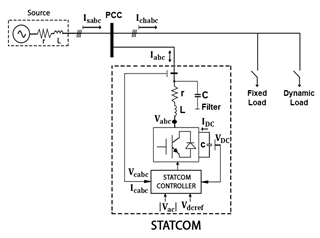Figure 1. Basic structure of a STATCOM coupled to the network

## 2.png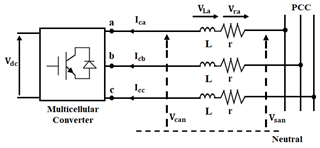Figure 2. STATCOM equivalent diagram

The major component of the system is the STATCOM compensator to regulate the voltage at the common connection point (PCC) between the grid and the loads.

As Figure 2 reveals, the STATCOM is presented with a block diagram. It consists of a three-phase multicellular converter with a capacitor on the direct current (DC) side and a coupling inductor on the alternating current (AC) side, and it is connected to the power grid. The STATCOM regulates the voltage at the PCC by controlling the amount of reactive power injected or absorbed into the grid.

The operating principle of STATCOM is simple. By varying the magnitude of the output voltages produced, the exchange of reactive power between the inverter and the AC system is controlled by the magnitude of the voltage produced at the inverter output. There are three possibilities in Figure 3. First, if the amplitude of the voltage produced by the inverter (Vcan) is higher than that of the AC system (Vsan), the reactive current (Ica) will be injected into the system and the inverter will behave as a source of reactive power. If (Vcan) is equal to the amplitude of the AC system voltage (Vsan), there will be no exchange of reactive power. Finally, if (Vcan) is lower than that of the AC system (Vsan), the reactive current (Ica) will be absorbed by the inverter.

As it is displayed in Figure 3 (b), when the current (Ica) lags behind the voltage (Vsan) by π/2, the device acts like a reactor, absorbing reactive power. When (Ica) leads the voltage (Vsan) by π/2, it acts like a capacitor, supplying reactive energy (Figure 3 (c)). If (Vsan) = (Vcan) the current goes through the reactance x is zero and there is no energy exchange (Figure 3 (a)) .

## 3.png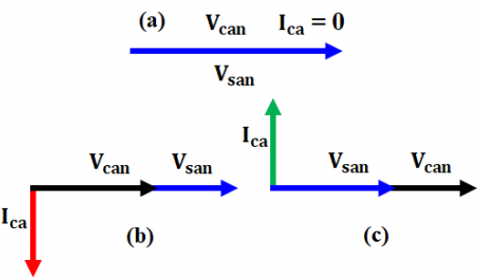Figure 3. STATCOM operating modes 

2.1 Converter model

In order to develop a model of the multicellular series converter, the following simplifying assumptions: perfect switches, perfect sources and neglected dead times are taken into consideration. The three-phase multicellular series converter fed by a DC voltage source is demonstrated.

Perfect switches: The switching of the switches is instantaneous (zero closing and opening time) and without losses. Finally, the voltage drop in the switches is considered zero in conduction.

Perfect sources: The voltage at the terminals of the DC bus is constant and does not vary with the power exchanged.

Neglected dead time: The aim of our study is to reduce the switching frequency, so the dead times will have little influence.

The difference between the real and simplified model (Figure 4) is to linearize the studied model.

## 4.png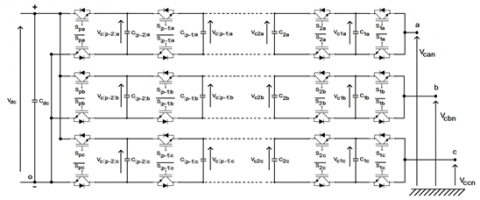Figure 4. Multicellular series converter

Table 1. Characteristic quantities of the combination of P switching cells

 Number of associated cells Number of associated capacitors Number of output voltage levels Possible output levels 2 3 4 P 1 2 3 P-1 3 4 5 P+1 0, Vdc/2 et Vdc 0, Vdc/3, 2Vdc/3 et Vdc 0, Vdc/4, 2Vdc/4, 3Vdc/4 et Vdc 0, Vdc/P, 2Vdc/P,…, Vdc

It is based on the series connection of p nested cells separated by (p -1) floating capacitors. Each cell contains two power switches controlled in a complementary way [19, 20].

The modeling objective is to find a relation between both of the control and electrical quantities for the converter's AC and DC parts.

The multicellular converter is controlled by switching functions Sik (K=a, b, c) denotes the phase and (i=1, 2, ...p denotes the cell concerned) whose value is between 0 and 1. If a switch is blocked, Sik = 0 and if it is conducting, then Sik = 1. Furthermore, when Sik = 1, then its complement $\bar{S}_{i k}$= 1, so as not to short-circuit an arm of the converter.

The cell voltages can be expressed in terms of the capacitor voltages:

$V_{\text {celik }}=V_{c i k}-V_{c(i-1) k}$      (1)

With  $V_{c 0 k}=0$ and $V_{c p k}=V_{d c}$.

Under steady state and equilibrium conditions, all cells have the same voltage level which is equal to:

$V_{\text {celik }}=V_{d c} / p$     (2)

Under these conditions the voltages across the capacitors take the values:

$V_{c i k}=i \times \frac{V_{d c}}{p}$     (3)

The output voltages VckO of the converter with respect to point O are therefore given by:

$V_{c k O}=\frac{V_{d c}}{p} \times \sum_{i=1}^{p} S_{i k}$     (4)

where:

VckO: voltages between point O (-) and converter output points of a, b, c phases;

S: control switch;

K = a, b, c: phase;

Vdc: direct voltage;

i= 1, 2, 3, ...p: cell concerned;

p: cells number in each phase;

The characteristic quantities of the combination of P switching cells are defined in the following Table 1.

The voltages Vckn = VckO –VnOVckn=VckO-VnO, (k=a, b, c), at the terminals of the load, with respect to the neutral point of potential VnO = (VaO + VbO + VcO)/3VnO=VaO+VbO+VcO/3, are written [21, 22]:

$\left[\begin{array}{c}V_{c a n} \\ V_{c b n} \\ V_{c c n}\end{array}\right]=\frac{1}{3}\left[\begin{array}{ccc}2 & -1 & -1 \\ -1 & 2 & -1 \\ -1 & -1 & 2\end{array}\right] \times\left[\begin{array}{c}V_{a o} \\ V_{b O} \\ V_{c o}\end{array}\right]$     (5)

It can be inferred from the analysis of Figure 2 that the STATCOM state space Eq. (6) are obtained, where Vsabc represents the grid voltages at the PCC, Vcabc is the three-phase output voltage from the multicellular converter, and Icabc is the three-phase balanced current flowing from the STATCOM [4, 23].

By applying Kirchhoff's second law (Law of meshes). We obtain the Eq. (6):

$\left\{\begin{array}{l}V_{s a n}=r I_{c a}+L \frac{d I_{c a}}{d t}+V_{c a n} \\ V_{s b n}=r I_{c b}+L \frac{d I_{c b}}{d t}+V_{c b n} \\ V_{s c n}=r I_{c c}+L \frac{d I_{c c}}{d t}+V_{c c n}\end{array}\right.$      (6)

Or:

$\left\{\begin{array}{l}V_{\text {san }}=V_{M} \cos (\omega t) \\ V_{\text {sbn }}=V_{M} \cos (\omega t-(2 \pi / 3)) \\ V_{\text {scn }}=V_{M} \cos (\omega t-(4 \pi / 3))\end{array}\right.$     (7)

Thus, the following equation defines the expression of the currents exchanged with the network:

$\frac{d}{d t}\left[\begin{array}{c}I_{c a} \\ I_{c b} \\ I_{c c}\end{array}\right]=\left[\begin{array}{ccc}-\frac{r}{L} & 0 & 0 \\ 0 & -\frac{r}{L} & 0 \\ 0 & 0 & -\frac{r}{L}\end{array}\right] \times\left[\begin{array}{c}I_{c a} \\ I_{c b} \\ I_{c c}\end{array}\right]$$+\frac{1}{L}\left[\begin{array}{lll}1 & 0 & 0 \\ 0 & 1 & 0 \\ 0 & 0 & 1\end{array}\right] \times\left[\begin{array}{c}V_{\text {san }}-V_{\text {can }} \\ V_{s b n}-V_{c b n} \\ V_{s c n}-V_{c c n}\end{array}\right] (8) The application of the Park transforms on the STATCOM model equations reveals a coupling term between the d-axis and q-axis component of the currents. \frac{d}{d t}\left[\begin{array}{c}I_{d} \\ I_{q}\end{array}\right]=\left[\begin{array}{cc}-\frac{r}{L} & 0 \\ 0 & -\frac{r}{L}\end{array}\right]\left[\begin{array}{c}I_{d} \\ I_{q}\end{array}\right]+\left[\begin{array}{cc}0 & \omega \\ -\omega & 0\end{array}\right]\left[\begin{array}{c}I_{d} \\ I_{q}\end{array}\right]$$+\frac{1}{L}\left[\begin{array}{ll}V_{s d} & V_{c d} \\ V_{s q} & V_{c q}\end{array}\right]$      (9)

The two equations provide the expressions for the active power P and reactive power Q exchanged between the network and the STATCOM .

$P=\frac{3}{2}\left(V_{s d} I_{d}+V_{s q} I_{q}\right)$     (10)

$Q=\frac{3}{2}\left(V_{s d} I_{q}-V_{s q} I_{d}\right)$     (11)

Since Vsq = 0Vsq=0, is based on the instantaneous active and reactive power definition, the two equations can be obtained as follows:

$P=\frac{3}{2} V_{s d} I_{d}$      (12)

$Q=\frac{3}{2} V_{s d} I_{q}$     (13)

2.2 STATCOM control

The detailed control system of STATCOM is shown in Figure 5. In this system, the error signal between the rms measured and the rms AC voltage reference values is given to a PI regulator, which produces a reference current Iqref. Similarly, the error between the measured and the DC voltage reference values is given to a PI regulator that produces a reference current Idref. The three-phase grid currents at the PCC are transformed into dq reference frame to create, Id and Iq. These currents are then compared to the corresponding reference values to create error signals (ΔId and ΔIq) which are transmitted to the PI controller in order to create the vectors of the reference voltages (Vsdref and Vsqref). Due to Park’s inverse transformation, the voltages Vsdref and Vsqref are converted to Vsaref, Vsbref and Vscref that are required by the SPWM generator [25-27].

To generate PWM control pulses for an N-level voltage converter, N-1 triangular carriers are required. These carriers have the same frequency fc and the same amplitude Ac as illustrated in Figure 6.

## 5.pngFigure 5. Block diagram of the STATCOM control strategy

## 6.png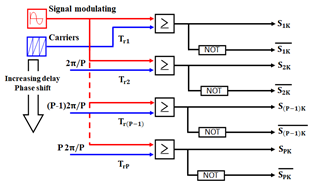Figure 6. Simplified diagram of the open loop PWM control of a multicellular converter

The pulse width modulation methods are classified according to the arrangement of the triangular carriers and the frequently utilized are [28, 29]:

- PS-PWM strategy (Phase Shifted Pulse Width Modulation):

In the case of a general n-level FCC, the carriers are phase shifted by 360°/(n-1) in order to provide optimal stepped multilevel output voltage waveforms as indicated in Figure 7.

## 7.png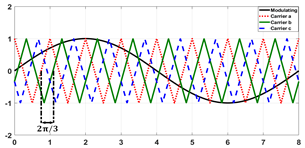Figure 7. PS-PWM modulation strategy

- CD-PWM strategy (Carrier Disposition Pulse Width Modulation):

There are three types of CD-PWM method. A distinction can be made between the strategies of:

• PD-PWM (Phase Disposition Pulse Width Modulation).
• POD-PWM (Phase Opposition Disposition Pulse Width Modulation).

• APOD-PWM (Alternative Phase Opposition Disposition Pulse Width Modulation).

Table 2. Simulation parameters 

 Architecture Modulation NPC FC H CD (PD, POD, APOD) PS PS

As it has the advantage of decreasing the switch frequency as well as allowing uniform discharge at the power sources, PS-PWM has been viewed as the most beneficial method for cascaded inverters. The choice of the technique PS-PWM is used in our work to control multicellular converters because it is:

- Simple to implement than other techniques;

- THD is low for all values of the modulation index;

- Also has a better THD than other techniques.

3. Simulation Results

We started by investigating the STATCOM’s efficiency in terms of reactive energy compensation and voltage regulation in a power grid. To this end, simulations have been carried out using the serial multi-cellular converters (three levels, four levels, five levels and six levels) based on phase shifted pulse width modulation (PS-PWM) control.

Table 3. Simulation parameters 

 Component Parameters Value AC Source Line-to-line rms voltage Frequency Source Resistance Source Inductance 381V 50Hz 7.3 m Ohm 0.23mH STATCOM DC-link Voltage L filter 750V 0.7mH Load Fixed load Dynamic load 50 kVA / +30kvar 10 kVA/ -50kvar

Two types of tests were applied in our model; in the first one, the fixed load is connected and in the second one, we connected the dynamic load. The entire system was simulated in MATLAB/Simulink with the parameters (Table 3).

In order to verify the validity of the proposed model, we carried out two experiments: one with STATCOM, and the other one without it.

3.1 Without STATCOM

The RMS voltage per phase, the three-phase voltages and currents at the common connection point (PCC) without STATCOM are displayed in Figure 8 and Figure 9 respectively. The inductive load during the interval [0s 1s] causes the voltage drop. Moreover, an overvoltage that is caused by the capacitive load during the interval [1s 1.3s]. [1.3s 1.5s] return to the initial state. As it is illustrated in Figure 10, inductive and capacitive loads destabilize the evolution of reactive power in the network resulting from the phase shift between the voltage and the current of the network.

## 8.png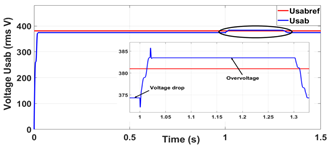Figure 8. RMS voltage between phases without STATCOM

## 9.png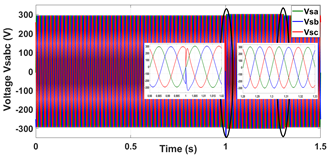Figure 9. Three-phase voltage (abc) of the source without STATCOM

## 10.png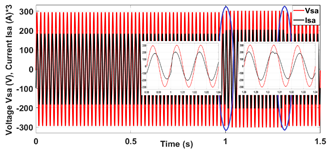Figure 10. Source voltage and current for phase (a) at PCC without STATCOM

3.2 With STATCOM

These tests are repeated in the presence of STATCOM at the common connection point (PCC) with the same loads. Figure 11 and Figure 12 reveal that the positive reaction of STATCOM manages to maintain the constant voltage at the common point of connection PCC by controlling the amount of reactive power injected or absorbed at the common point of connection (PCC).

During the interval of 0s to 1s the STATCOM injects a quantity of reactive power to maintain voltage levels at the common connection point (PCC). In that case, the compensator operates in capacitive mode. On the other hand, STATCOM, in the interval 1s to 1.3s, absorbs a quantity of reactive power that maintains the voltage levels in the line, and therefore; the compensator works in the inductive mode as shown in Figure 13.

## 11.png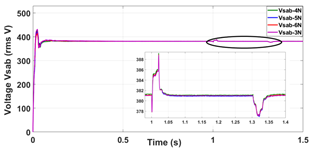Figure 11. RMS voltage between phases at PCC with STATCOM

## 12.png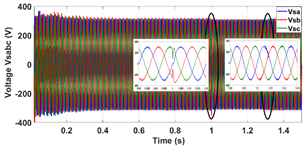Figure 12. Three-phase voltage (abc) of the source with STATCOM

We use two load types to examine STATCOM's behavior in the inductive and capacitive modes and to observe its behavior when the modes change (positive feedback).

## 13.png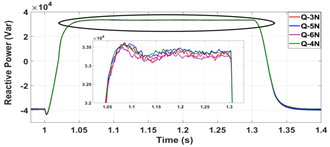Figure 13. Reactive power observed/supplied by STATCOM

Figure 14 displays that this reactive power exchange is realized through the AC filter leakage inductance which smoothing the currents in advance or in delay of the angle (π/2) with the converter output voltages imposed to grid.

## 14.png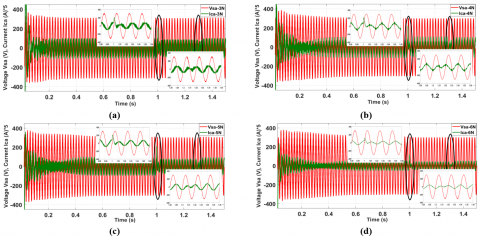Figure 14. Source voltage and current waveform of STATCOM output

The curve in Figure 15 clearly demonstrates the effectiveness of the block control of DC voltage in maintaining a constant voltage across the capacitor. This can be done by the good tunning of $K_{p}$ and $K_{i}$ (Figure 5).

## 15.png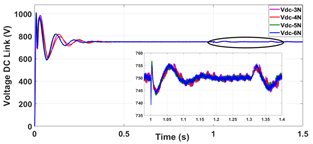Figure 15. Response curves of DC voltage

## 16.png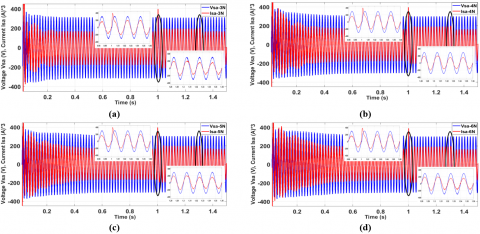Figure 16. Source voltage and current with STATCOM

Figure 16 exhibits phase (a) voltage and current waveforms after compensation. This reflects a tight correspondence between voltage and current phases. The power factor jumps from 0.875 to 0.99.

Figure 17 shows the voltage curves with floating capacities and the DC-bus voltages of the three, four, five and six levels multicellular converters.

It can be noticed from the figures how the floating arm voltages follow their references with a small oscillation observed on the curves.

## 17.png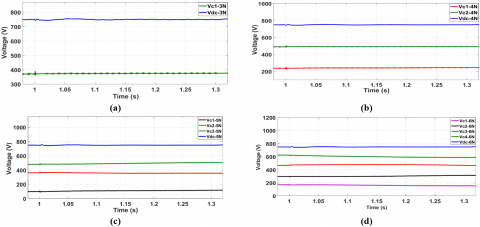Figure 17. Voltage curves with floating capacities and DC voltage

Figure 18 and Figure 19 depict the voltages between   phase (a) and neutral point O (Vcao), as well as the voltages between phase (Vcab) at the multicellular converters output with levels three, four, five and six.

This section suggests a comparative analysis with simulation results obtained in four studied STATCOM models.

The overall simulation results are summarized in Table 4, which presents the performance of multicellular STATCOMs on THD reduction.

## 18.png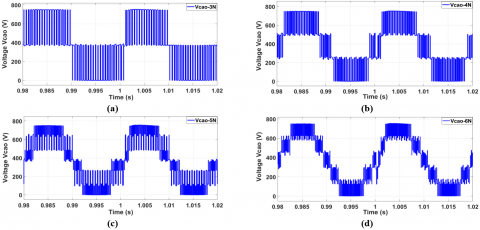Figure 18. Output voltage of the STATCOM converter (Vcao)

## 19.png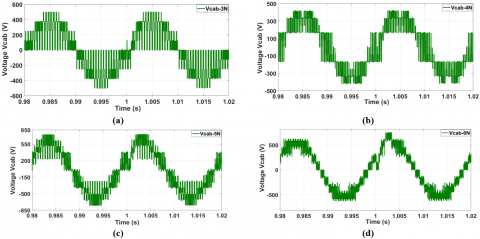Figure 19. Output voltage between phases of the STATCOM converter (Vcab)

## 20.png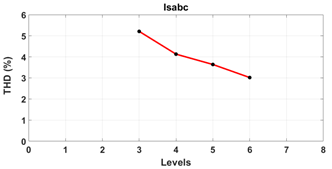Figure 20. THD for levels PS-PWM current at PCC

Table 4. Comparative analysis

 Line current Isabc Line voltage Vcab Phase Voltage Van 3N 4N 5N 6N THD% 5.21 4.13 3.64 3.02 FD 60.54 61.10 61.02 60.41 THD% 64.14 41.35 34.05 22.24 FD 536.4 535.8 536.1 536 THD% 64.40 41.21 34.58 22.23 FD 309.6 309.6 309.6 309.6

## 21.png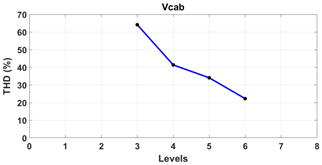Figure 21. THD for levels PS-PWM output voltage between phases

The previous table showed that the four types of compensators, controlled in the same way, provide almost identical values of current load and phase-to-phase voltages at the converter output. Therefore, as the number of levels increases, the harmonics are lower.

Figure 20 and Figure 21 provides a global perspective on THD reduction performance of multilevel inverters.

A decrease of the THD from level three to six is noticed. It can be indicated that as the number of levels increases, the harmonics become weaker, which guarantee a low THD below the IEEE-519 standard (5%). Here multilevel converters implementation gains momentum.

4. Conclusions

This study demonstrated the modeling and performance analysis of multicellular converters (three to six levels) used in STATCOM applications to voltage regulation, reactive energy compensation, and power factor improvement in a power system. A proposed STATCOM mathematical model was analyzed in detail for designing an appropriate control.

Furthermore, PWM control based on phase shifted allowed floating capacitor voltages to be equilibrated, and consequently, enhancing the output voltage signal and minimizing the current THD.

STATCOM model and control were implemented on MATLAB /SIMULINK environment to investigate the steady state and dynamic performance. A conclusion which can be underlined on the basis of the obtained results is that using the proposed control, STATCOM is efficient in supplying or absorbing the reactive energy required for maintaining a stable voltage at the common connection point (PCC) regardless of disturbance type.

The findings also suggest that this approach could be useful to provide a basis for a STATCOM experimental setup validation based on the serial multicellular converter.

Nomenclature
 STATCOM Static Synchronous Compensator PS-PWM Phase Shifted Pulse Width Modulation SPWM Sinusoidal pulse width modulation PCC Point Common Connection RMS Root Mean Square THD Total harmonic distortion FD Fundamental
References

 Hingorani, N.G., Gyugyi L. (1999). Understanding FACTS: Concepts and technology of Flexible AC Transmission Systems. Wiley-IEEE Press, ISBN: 978-0-780-33455-7.

 Padiyar, K.R. (2007). FACTS controllers in power transmission and distribution. Department of Electrical Engineering, Indian Institute of Science, ISBN: 978-81-224-2541-3.

 Morkhade, C.E., Bhushan, S.R. (2013). Improvement in voltage profile using FACT device. International Journal of Scientific & Engineering Research, 4(6): 27-32.

 Crappe, M. (2006). Exploitation des Réseaux Electriques avec L’électronique de Puissance. Hermès science publications-Lavoisier, ISBN: 978-2-7462-1198-8.

 Huang, Z., Shengzhen, Y., Hongyan, Y., Xingming, F. (2012). A control method for SVPWM-based STATCOM using active-reactive current decouple under DQ coordinate system. International Conference and Exposition on Electrical and Power Engineering (EPE), 25-27 October, Iasi, Romania, pp. 191-196. https://doi.org/10.1109/ICEPE.2012.6463929

 Murugesan, K., Senthil Kumaran, M., Anitha Roseline, J., Vijayenthiran, S., Kubera Murthi, M., Maheswari, A.C. (2019). DSTATCOM using matrix converter for reactive power compensation. 2019 Fifth International Conference on Electrical Energy Systems (ICEES), pp. 1-6. https://doi.org/10.1109/ICEES.2019.8719322

 Barik, S.R., Nayak, B., Dash, S. (2014). A comparative analysis of three level VSC based multi-pulse STATCOM. International Journal of Engineering and Technology, 6(3): 1550-1563.

 Onah, A.J. (2018). A three-level inverter based static compensator (STATCOM). European Journal of Electrical Engineering and Computer Science, 2(5): 1-7. https://doi.org/10.24018/ejece.2018.2.5.28

 Farhadi-Kangarlu, M., Torshakaan, M.B. (2018). Multilevel inverter based distribution STATCOM: Application of the cross-switched multilevel inverter. 26th Iranian Conference on Electrical Engineering (ICEE), pp. 1144-1147. https://doi.org/10.1109/ICEE.2018.8472502

 Shahnia, F., Rajakaruna, S., Ghosh, A. (2014). Static Compensators (STATCOMs) in Power Systems. Springer, Singapore. https://doi.org/10.1007/978-981-287-281-4

 Baldé, M., Doumbia, M.L., Chériti, A., Benachaiba, C. (2011). Comparative study of NPC and cascaded converters topologies. Renewable Energy and Power Quality Journal, 9: 185-190. https://doi.org/10.24084/repqj09.282

 Liu, X., Lv, J., Gao, C., Chen, Z., Chen, S. (2016). A novel STATCOM based on diode-clamped modular multilevel converters. IEEE Transactions on Power Electronics, 32(8): 5964-5977. https://doi.org/10.1109/TPEL.2016.2616495

 Belakehal, S., Djellad, A., Chenni, R. (2019). Performance comparison of multicell series and NPC multilevel converters for a STATCOM. Electrical Engineering & Electromechanics, 5: 60-67. https://doi.org/10.20998/2074-272X.2019.5.10

 Sadigh, A.K., Dargahi, V., Corzine, K.A. (2015). Analytical determination of conduction and switching power losses in flying-capacitor-based active neutral-point-clamped multilevel converter. IEEE Transactions on Power Electronics, 31(8): 5473-5494. https://doi.org/10.1109/TPEL.2015.2498107

 Sadigh, A.K., Dargahi, V., Corzine, K. (2016). Investigation of conduction and switching power losses in modified stacked multicell converters. IEEE Transactions on Industrial Electronics, 63(12): 7780-7791. https://doi.org/10.1109/TIE.2016.2607160

 Oghorada, O.J.K., Zhang, L., Esan, B.A., Dickson, E. (2019). Carrier-based sinusoidal pulse-width modulation techniques for flying capacitor modular multi-level cascaded converter. Heliyon, 5(12): e03022. https://doi.org/10.1016/j.heliyon.2019.e03022

 Mihalic, R., Eremia, M., Blazic, B. (2016). Static synchronous compensator–Statcom. Advanced Solutions in Power Systems: HVDC, FACTS, and Artificial Intelligence: HVDC, FACTS, and Artificial Intelligence, pp. 459-525. https://doi.org/10.1002/9781119175391.ch8

 Wildi, T. (2002). Electrical Machines, Drives, and Power Systems. Fifth Edition, Pearson Education, Upper Saddle River, New Jersey, ISBN: 0-13-093083-0.

 Laamiri, S., Ghanes, M., Santomenna, G. (2019). Observer based direct control strategy for a multi-level three phase flying-capacitor inverter. Control Engineering Practice, 86: 155-165. https://doi.org/10.1016/j.conengprac.2019.03.011

 Aguilera, R.P., Lezana, P., Quevedo, D.E. (2015). Switched model predictive control for improved transient and steady-state performance. IEEE Transactions on Industrial Informatics, 11(4): 968-977. https://doi.org/10.1109/TII.2015.2449992

 Amet, L., Ghanes, M., Barbot, J.P. (2012). Commande directe d'un convertisseur multicellulaire: résultats exp erimentaux. In CIFA-7ème Conférence Internationale Francophone d'Automatique-2012.

 Rathore, K., Kumar D., Yadav, S. (2015). D-STATCOM modeling and simulation applying control schemes for power quality improvement. International Journal of Electrical and Electronic Engineering & Telecommunications, 4(2): 27-36.

 Xu, Y., Li, F. (2014). Adaptive PI control of STATCOM for voltage regulation. IEEE Transactions on Power Delivery, 29(3): 1002-1011. https://doi.org/10.1109/TPWRD.2013.2291576

 Schauder, C., Mehta, H. (1993). Vector analysis and control of advanced static VAR compensators. IEE Proceedings C Generation, Transmission and Distribution, 140(4): 299-306. https://doi.org/10.1049/ip-c.1993.0044

 Wanchai, S. (2016). Design and analysis three phase three level diode-clamped grid connected inverter. Energy Procedia, 89: 130-136. https://doi.org/10.1016/j.egypro.2016.05.019

 Murugesan, K., Muthu, R. (2011). Modeling and simulation of DSTATCOM for voltage regulations. 1st International Conference on Electrical Energy Systems, pp. 1-5. https://doi.org/10.1109/icees.2011.5727975

 Giroux, P., Sybille, G., Le-Huy, H. (2001). Modeling and simulation of a distribution STATCOM using Simulink's Power System Blockset. In IECON'01. 27th Annual Conference of the IEEE Industrial Electronics Society (Cat. No. 37243), 2: 990-994. https://doi.org/10.1109/iecon.2001.975905

 Radan, A., Shahirinia, A.H. (2008). Novel carrier-based PWM methods for mu1ti-level inverters. EPE Journal, 18(2): 16-21. https://doi.org/10.1080/09398368.2008.11463677

 Ghias, A.M. (2014). On Performance Advances of Flying Capacitor Multilevel Converter Topologies. PhD Thesis, The University of New South Wales, Australia.

 Berkoune, K. (2016). Approche mathématique pour la modulation de largeur d'impulsion pour la conversion statique de l'énergie électrique: application aux onduleurs multiniveaux (Doctoral dissertation, Université Paul Sabatier-Toulouse III).

 Deniz, E., Tuncer, S., Gencoglu, M.T. (2009). Voltage regulation in a distribution system by using STATCOM with three level diode clamped inverter. In IATS’09 5th-International Advanced Technologies Symposium, pp. 1-7.